# TI-36X Pro scientific calculator

Advanced, four-line scientific calculator, with higher-level math and science functionality. Ideal for computer science and engineering courses in which graphing technology may not be permitted.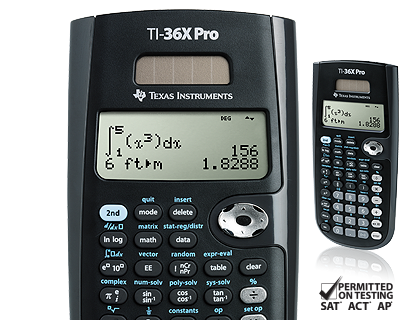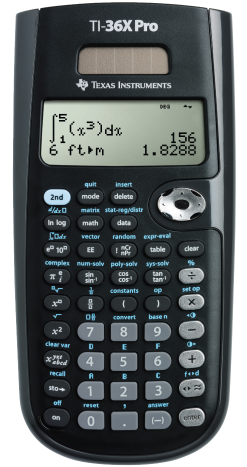## Key features

• Four-line display
• One- and two-variable statistics
• MultiView™ display shows multiple calculations at the same time on screen
• Select degrees/radians, floating/fix, number format modes
• Choose from three solvers: numeric equation, polynomial and system of linear equations
• Display a defined function in a tabular form
• Determine the numeric derivative and integral for real functions
• Perform vectors and matrices using a vector and matrix entry window

Ideal for:

• Algebra I and II
• Geometry
• Trigonometry
• Statistics
• Calculus
• Biology
• Chemistry
• Physics
• Computer science
• College math
• College science
• College engineering

Exam acceptance
The TI-36X Pro scientific calculator is approved for use on SAT®, ACT®, and AP® exams.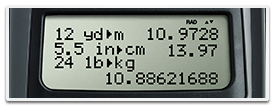## View multiple calculations at the same time

Compare results and explore patterns on-screen with the MultiView™ display, which supports up to four lines.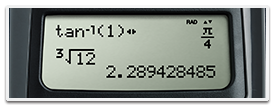## See math exactly as it appears in textbooks

See math expressions, symbols and stacked fractions exactly the way they appear in textbooks — no need to adapt to a technical syntax; also provides quick access to frequently used functions.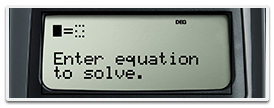## Built-in solvers

Intuitive prompts allow one to easily solve numeric equation, polynomial and system of linear equations.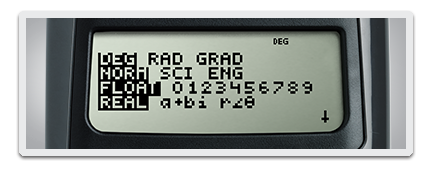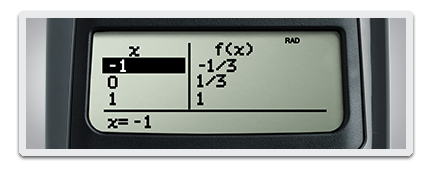## Explore (x,y) table of values

Students can easily explore an (x,y) table of values for a given function, automatically or by entering specific x values.

SAT® and AP® are trademarks registered by the College Board. ACT is a registered trademark of ACT, Inc. Neither are affiliated with, nor endorse, TI products. Policies subject to change. Visit www.collegeboard.org and www.act.org.## TI-36X Pro scientific calculator specifications#### Built-in functionality

• Review and edit previous entries via a scrollable home screen
• Paste inputs or outputs into new calculations
• MathPrint™ feature entry and output mode for viewing calculations in math notation, including answers in terms of pi, square roots and fraction
• Three solvers: numeric equation, polynomial and system of linear equations
• Numeric derivative and integral for real functions
• Vectors and Matrices
• Symbolic notation of π
• Toggle key to change the form of answers between exact and decimal approximation
• Stacked Fractions and Fraction functions
• Fraction/decimal/percent conversions
• Change between improper fractions and mixed numbers
• Automatic simplification of fractions
• Random number and random integer generator
• Central MODE menu for selecting calculator mode settings
• Functions accessed directly through keys or through pull-down menus
• Negation key
• One constant operator feature
• Combinations and permutations
• Trigonometry
• Hyperbolics
• Logs and antilogs
• %, x², ¹/x, yˆx, π, x!
• Fixed decimal capability
• (x,y) Table feature with Auto and Ask-x options
• Basic Data/List Editor with three lists
• List Formulas
• One- and two-variable statistics with permanent stat variable input storage
• EOS (Equation Operating System)
• Nine physical constants
• Eighteen metric/English conversions
• Up to eight pending operations
• Up to 23 levels of parentheses
• Error recovery capability
• Auto Power Off
• Quick/easy reset of calculator via two-key press or menu for exam purposes
• Eight memory variables (x, y, z, t, a, b, c, d)
• Scientific and engineering notation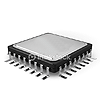#### Hardware

• Four-line × 16-character, easier-to-read LCD display
• Battery powered with solar cell assistance to lengthen battery life
• Auto Power Off
• Hard plastic, color-coded keys
• Non-skid rubber feet
• Impact-resistant cover with quick-reference card
• Snap-on protective hard case